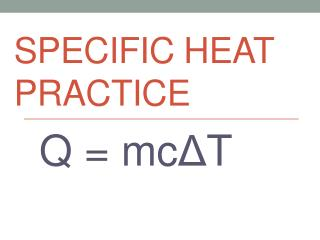DownloadDownload PresentationSpecific Heat Practice

# Specific Heat Practice

Download Presentation## Specific Heat Practice

- - - - - - - - - - - - - - - - - - - - - - - - - - - E N D - - - - - - - - - - - - - - - - - - - - - - - - - - -
##### Presentation Transcript

1. Specific Heat Practice Q = mcΔT

2. Problem #1 • How many joules of heat are needed to raise the temperature of 40.0 g of aluminum from 20°C to 58°C, if the specific heat of aluminum is 0.90 J/g°C?

3. Problem #2 • When a 140 g sample of aluminum (Al) absorbs 9612 J of energy, its temperature increases from 25oC to 105oC. Find the specific heat of aluminum.

4. Problem #3 • A 19.75-g piece of iron absorbs 1086.75 joules of heat energy, and its temperature changes from 25°C to 155°C. Calculate the specific heat capacity of iron

5. Problem #4 • A certain mass of water was heated with 41,870 Joules, raising its temperature from 21.0 °C to 38.5 °C. Find the mass of the water, in grams. (Cp of H2O = 4.184 J/g °C)

6. Problem #5 • How many joules of heat are needed to change 60.0 grams of ice at -12.0 °C to steam at 120.0 °C? • (Cp of H2O = 4.184 J/g °C)

7. Problem #6 • A certain mass of water was heated with 41,870 Joules, raising its temperature from 21.0 °C to 38.5 °C. Find the mass of the water, in grams. (Cp of H2O = 4.184 J/g °C)

8. Problem #7 • Calculate the number of joules given off when 37.0 grams of steam cools from 120.0 °C to ice at -20.0 °C. • (Cp of H2O = 4.184 J/g °C)

9. Problem #8 • The specific heat of ethanol is 2.46 J/g oC. Find the heat required to raise the temperature of 173 g of ethanol from 19oC to 45oC

10. Practice #8 • The specific heat of aluminum is 0.88 J/g ⁰C. How many joules will it take to make the temperature of a 50 g nugget to go up from 20 ⁰C to 70⁰C?

11. Practice #9? • How much heat is needed to warm 0.52 kg of gold from 30 ⁰C to 120 ⁰C? (cp = 136 J/kg ⁰C)

12. Practice #10 • If it takes 820 Joules of heat to warm a sample of zinc from 0 ⁰C to 50 ⁰C, what would be the mass of zinc? (cp= 380 J/kg ⁰C)

13. Practice #11 • What is the specific heat of silver if an 80.0 g sample is heated from 24.0 ⁰C to 49.0 ⁰C by adding 468.2J?

14. Practice #12 • The specific heat of iron is 0.46 J/g ⁰C. How many joules of heat will it take to make the temperature of a 150 g bar go up from 25 ⁰C to 60 ⁰C?

15. Practice #13 • What is the specific heat of copper if a 75 g sample is heated from 20 ⁰C to 24 ⁰C by adding 117 J?

16. Practice #14 • A 9.5 kg copper sculpture heats up during the day from 24 ⁰C to 78 ⁰C. How much energy was absorbed? (cp = 390 J/kg ⁰C)

17. Practice #15 • What would be the specific heat of a material that takes 310 joules of energy to warm 40 kg from 50 ⁰C to 65 ⁰C?